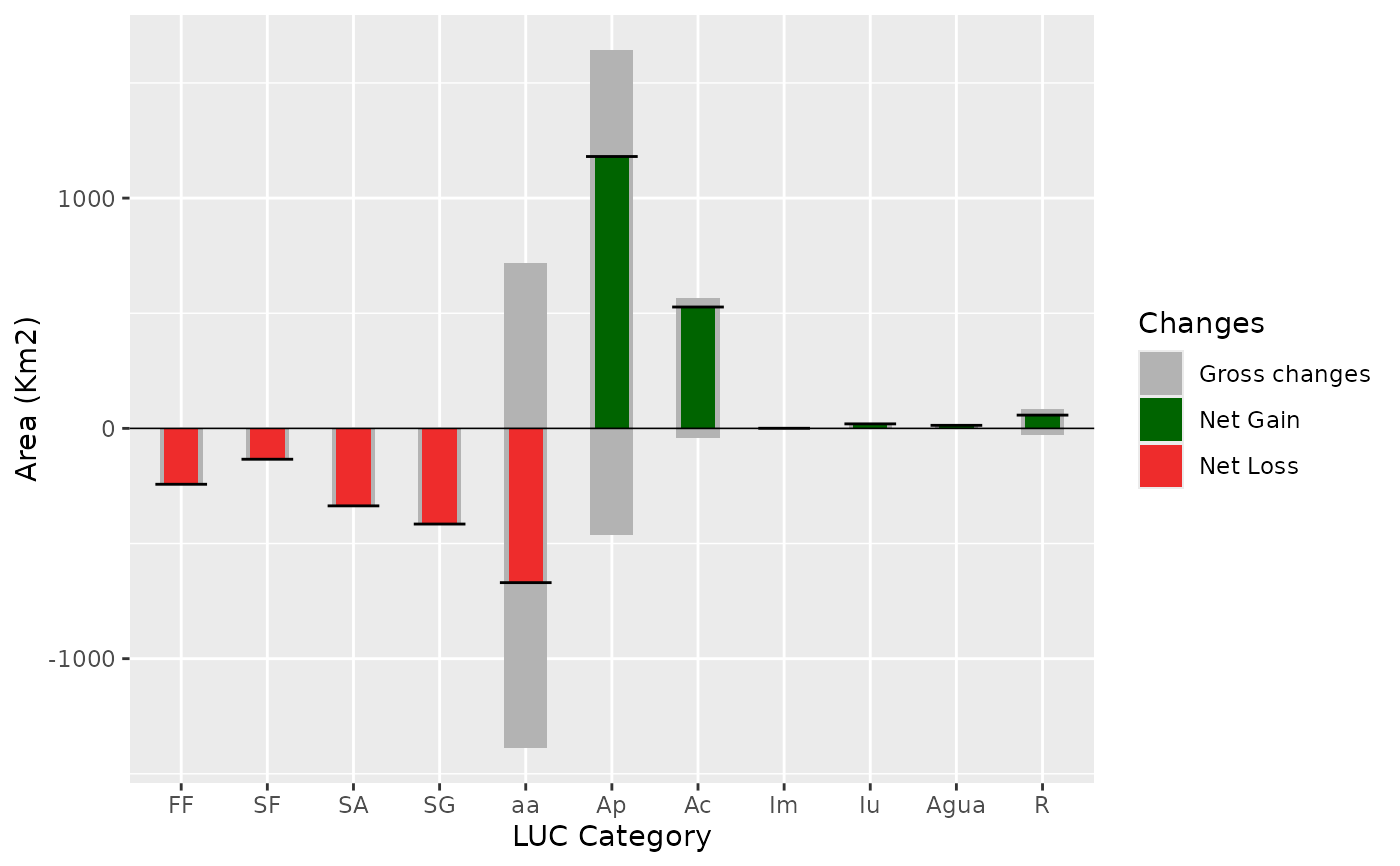A stacked barplot showing net and gross changes of LUC categories during the entire analysed time period.

netgrossplot(
dataset,
legendtable,
title = NULL,
xlab = "LUC category",
ylab = "Area (Km2)",
legend_title = "Changes",
changesLabel = c(GC = "Gross change", NG = "Net gain", NL = "Net loss"),
color = c(GC = "gray70", NG = "#006400", NL = "#EE2C2C"),
area_km2 = TRUE
)

## Arguments

dataset A table of the multi step transition (lulc_Mulstistep) generated by contingencyTable. A table containing the LUC legend items and their respective color (tb_legend). character. The title of the plot (optional), use NULL for no title. character. Label for the x axis. character. Label for the y axis. character. The title of the legend. character. Labels for the three types of changes, defaults are c(GC = "Gross change", NG = "Net gain", NL = "Net loss"). character. A vector defining the three bar colors. logical. If TRUE the change is computed in km2, if FALSE in pixel counts.

A bar plot

## Examples


# editing the category names

SL_2002_2014$tb_legend$categoryName <- factor(c("Ap", "FF", "SA", "SG", "aa", "SF",
"Agua", "Iu", "Ac", "R", "Im"),
levels = c("FF", "SF", "SA", "SG", "aa", "Ap",
"Ac", "Im", "Iu", "Agua", "R"))

# the plot
netgrossplot(dataset = SL_2002_2014$lulc_Multistep, legendtable = SL_2002_2014$tb_legend,
title = NULL,
xlab = "LUC Category",
changes = c(GC = "Gross changes", NG = "Net Gain", NL = "Net Loss"),
color = c(GC = "gray70", NG = "#006400", NL = "#EE2C2C"))